# Intermediate Maths Solutions for Inverse Trigonometric Functions Exercise 8(a)

Inter Maths 1A Exercise 8(a) Inverse  Trigonometric Functions text book  Solutions are given.

These solutions are very easy to understand.

Study the text book lesson very well. Observe the problems and solutions given in the text book. Try them well.

Observe the given solutions and try them in your own method.

# Inter Maths Solutions for Inverse Trigonometric Functions Exercise 8(a)

You can see the solutions for text book Maths 1A

Functions

Exercise 1(a)

Exercise 1(b)

Exercise 1(c)

Mathematical Induction

Exercise 2(a)

Matrices

Exercise 3(a)

Exercise 3(b)

Exercise 3(c)

Exercise 3(d)

Exercise 3(e)

Exercise 3(f)

Exercise 3(g)

Exercise 3(h)

Exercise 3(i)

Exercise 4(a)

Exercise 4(b)

Product of vectors

Exercise 5(a)

Exercise 5(b)

Exercise 5(c)

Trigonometric Ratios upto Transformations

Exercise 6(a)

Exercise 6(b)

Exercise 6(c)

Exercise 6(d)

Exercise 6(e)

Exercise 6(f)

Trigonometric Equations

Exercise 7(a)

Inverse Trigonometric Equations

Exercise 8(a)

Hyperbolic Functions

Exercise 9(a)

Properties of Triangles

Exercise 10(a)

Exercise 10(b)

Inter Maths Trigonometry text book solutions

Maths 1B solutions for

Locus

Exercise 1(a)

Transformation of Axes

Exercise 2(a)

Three Dimensional Coordinates

Exercise 5(a)

Exercise 5(b)

Direction Cosines and Direction Ratios

Exercise 6(a)
Exercise 6(b)
The Plane

Exercise 7(a)

Limits and Continuity

Exercise 8(a)

Exercise 8(b)

Exercise 8(e)

M

## Maths 1A Solutions Inter Exercise 8(a) Inverse Trigonometric Functions

Exercise 8(a)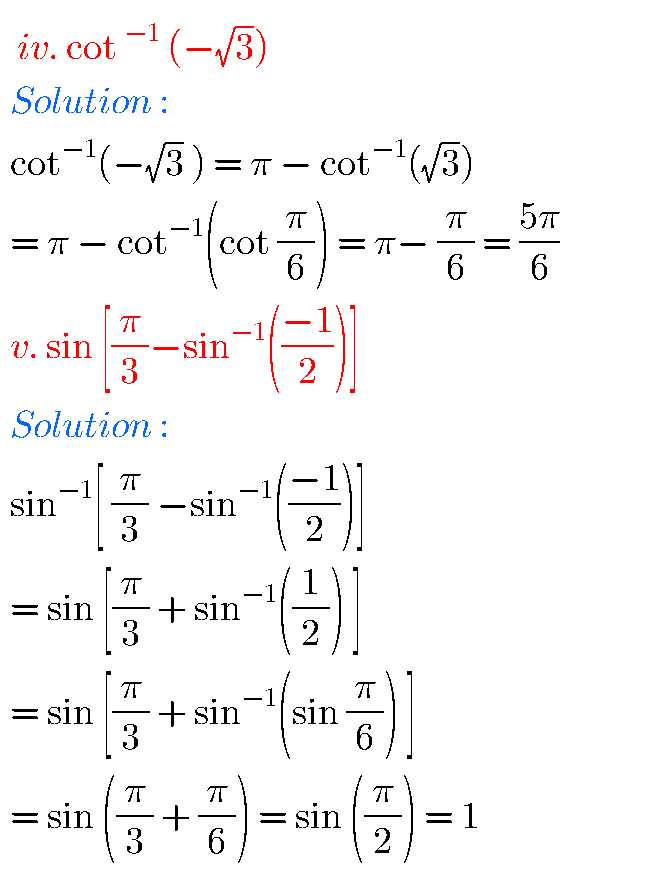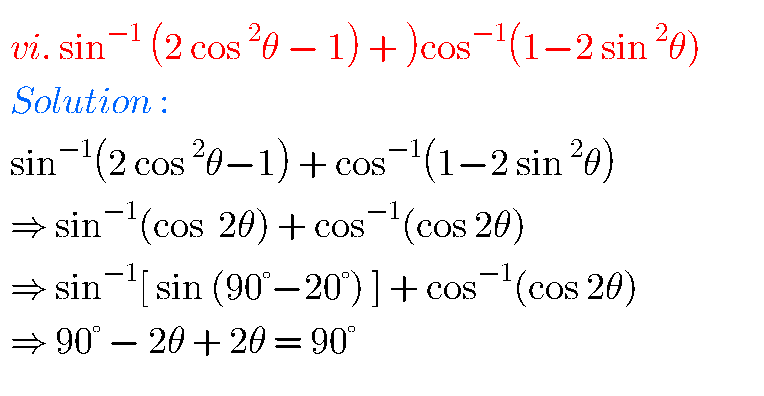## Inverse Trigonometric Functions Exercise 8(a) Solutions Inter Maths 1A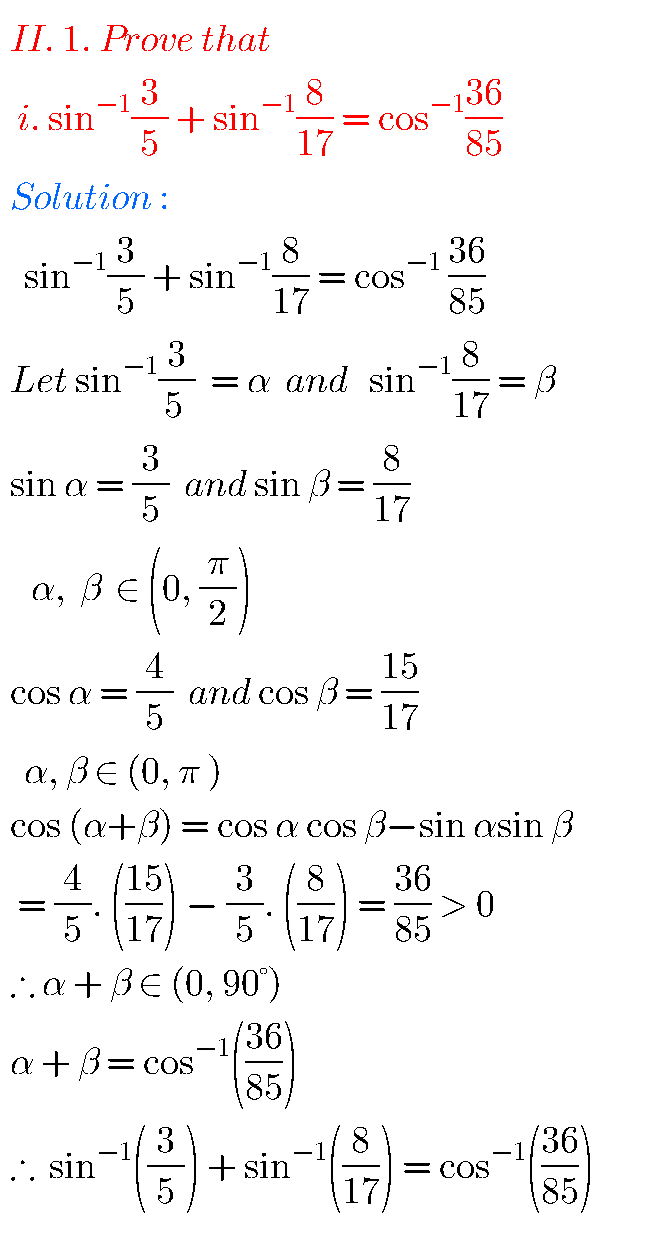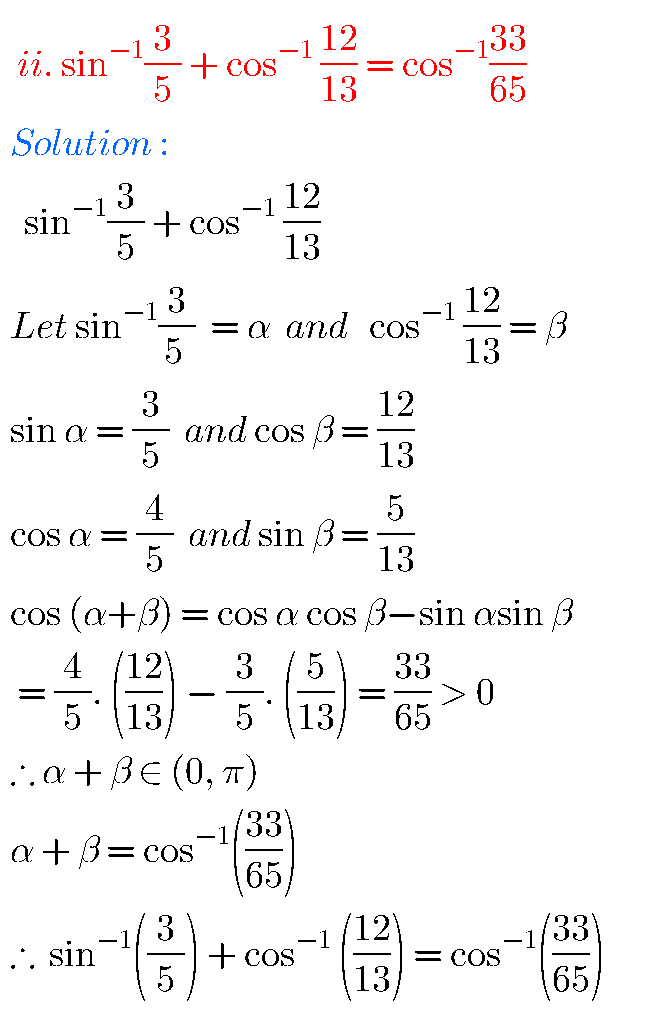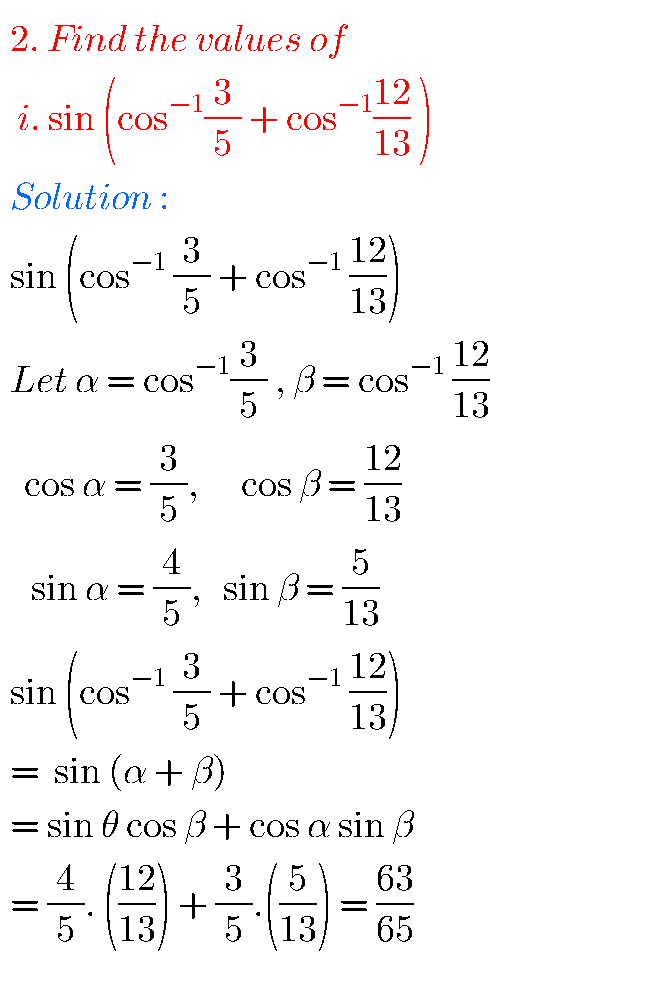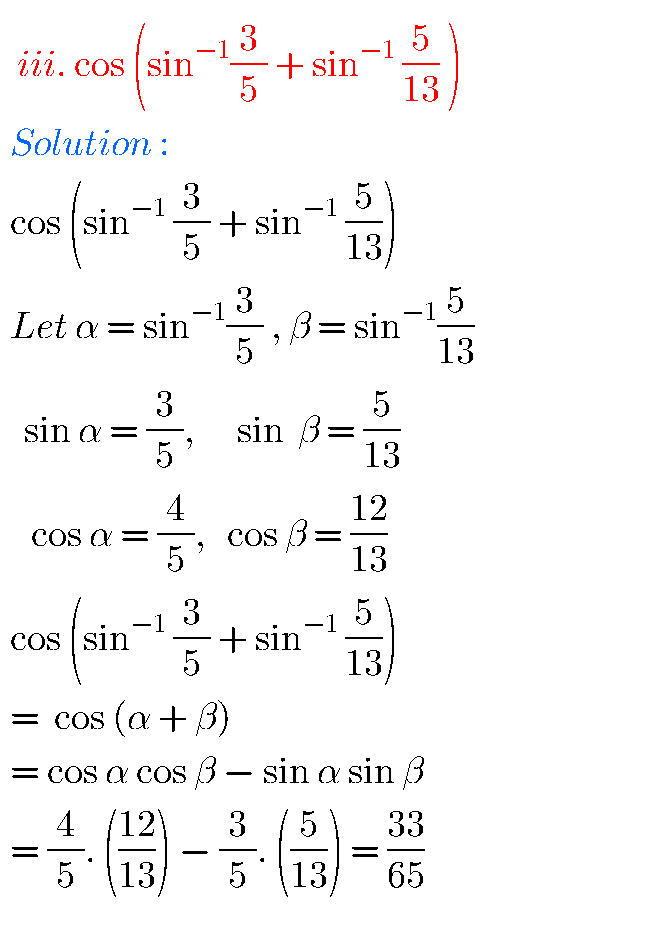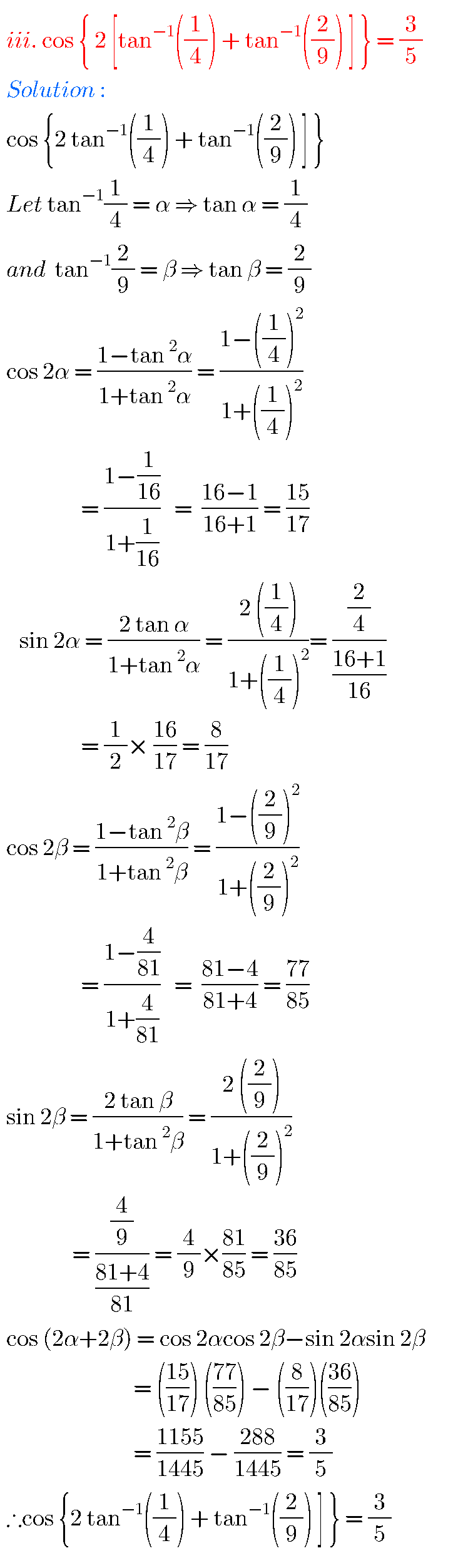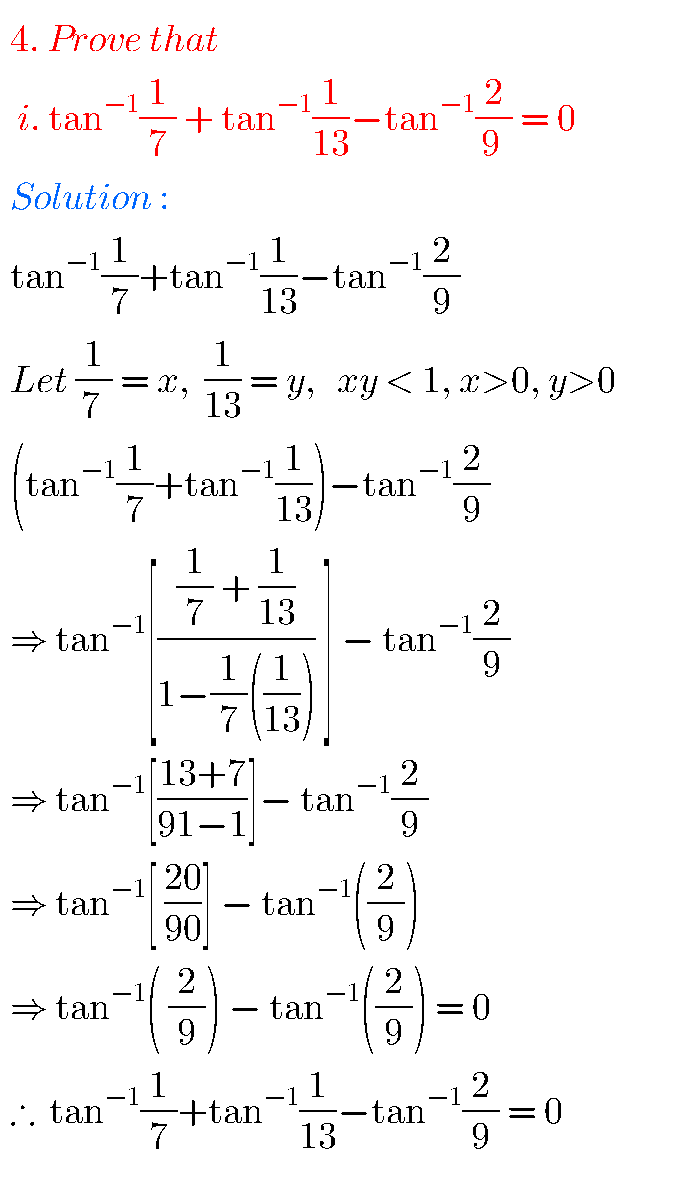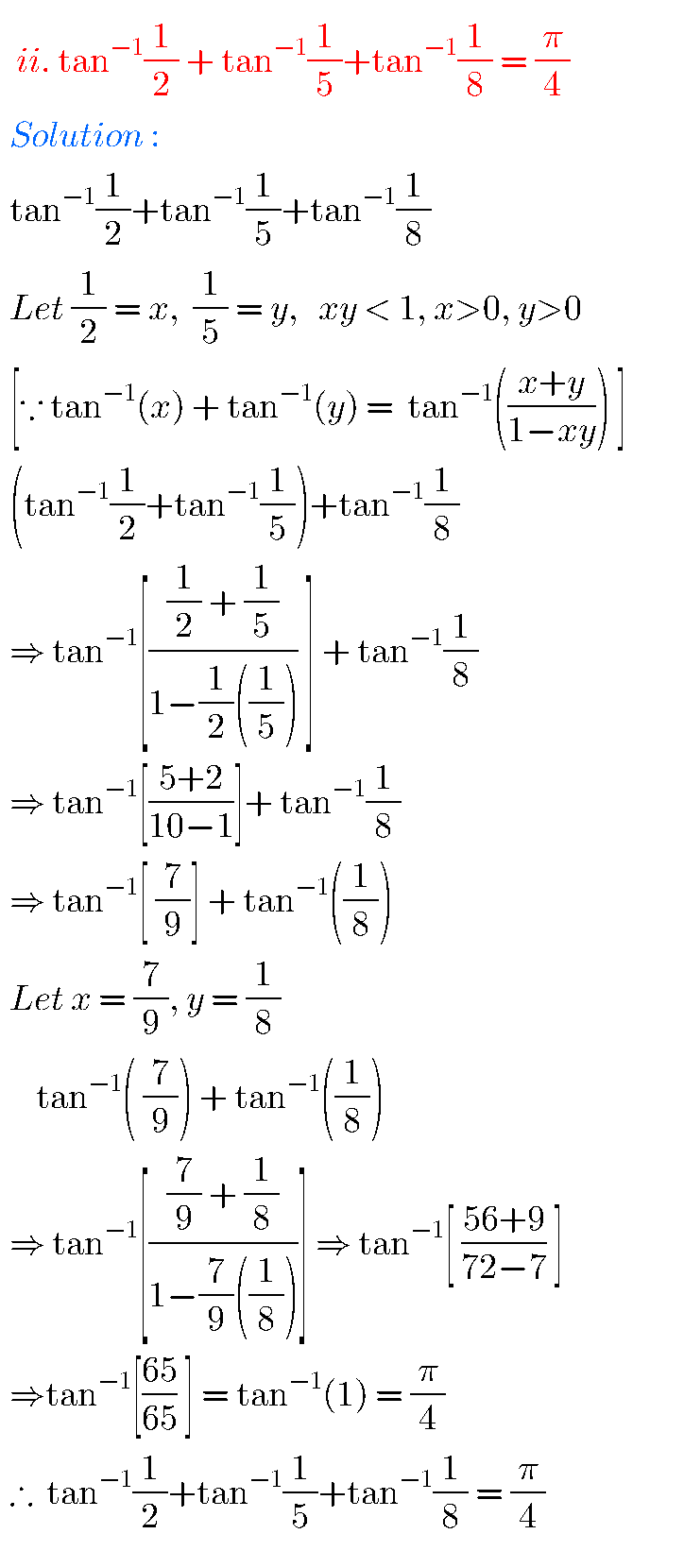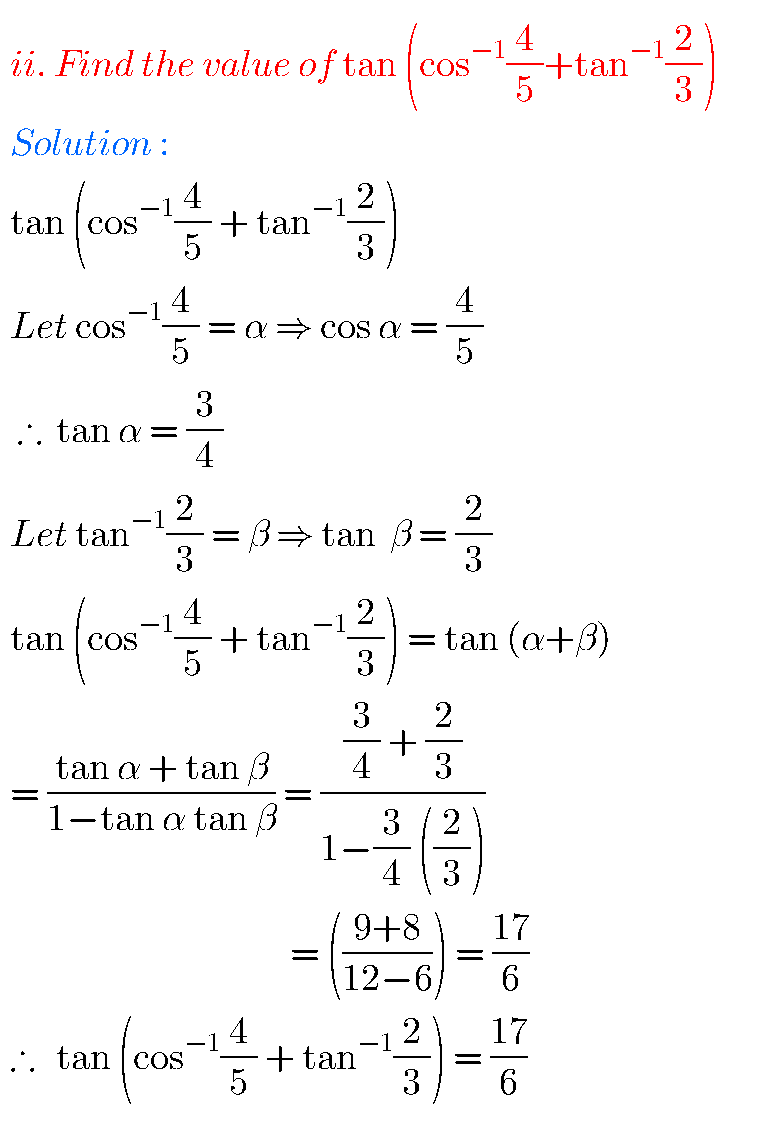### Exercise 8(a) Inverse Trigonometric Functions text book solutions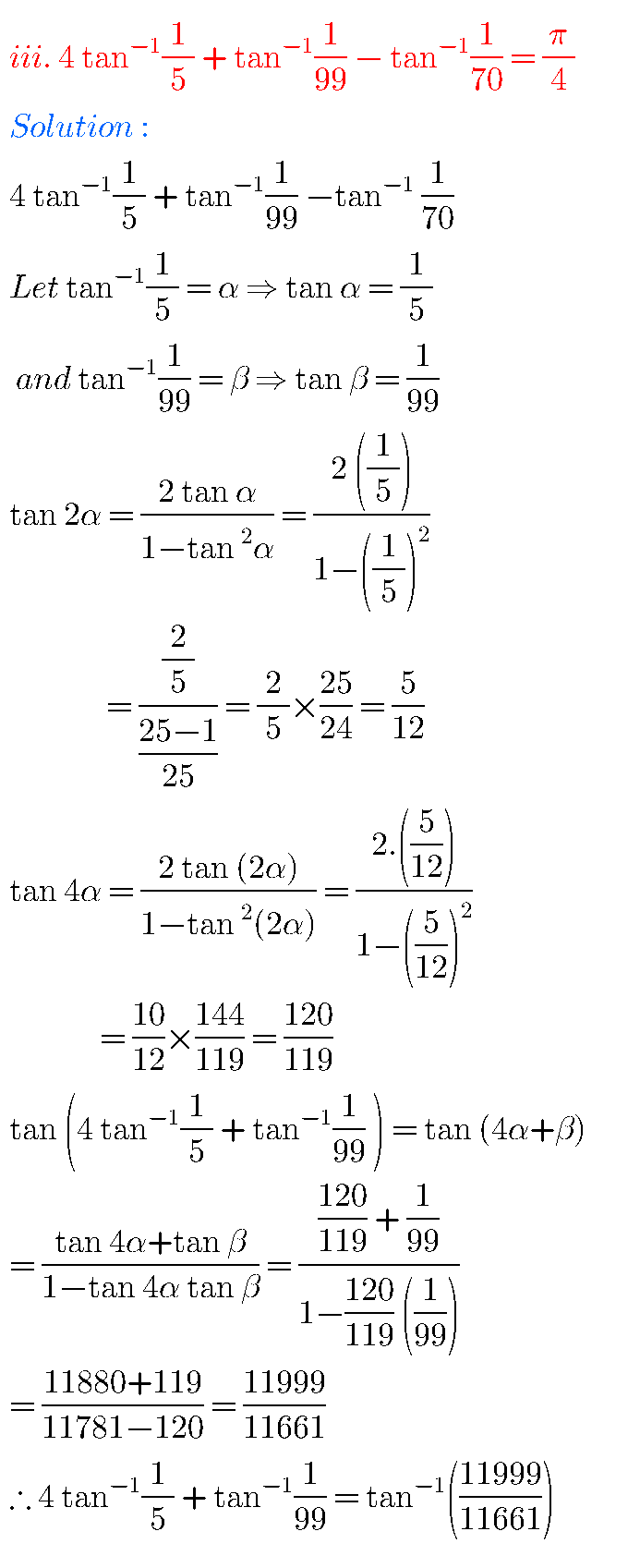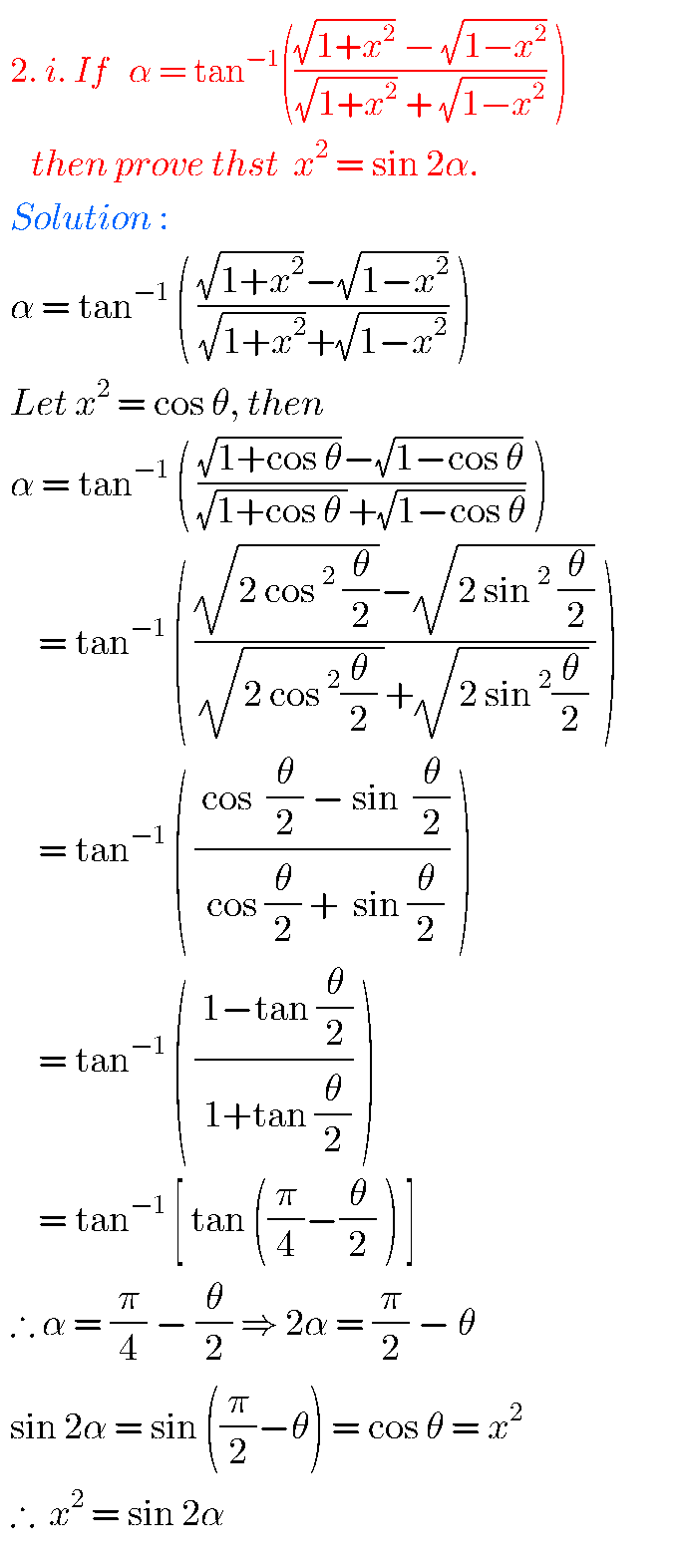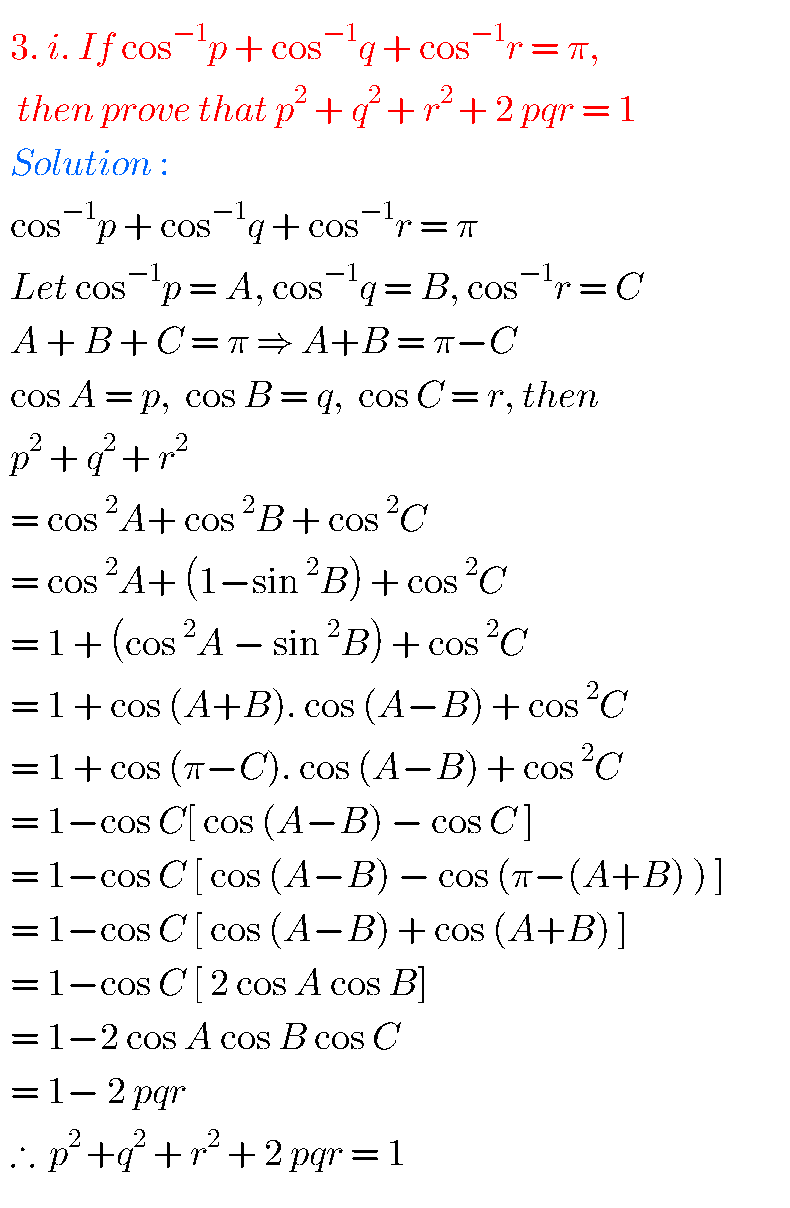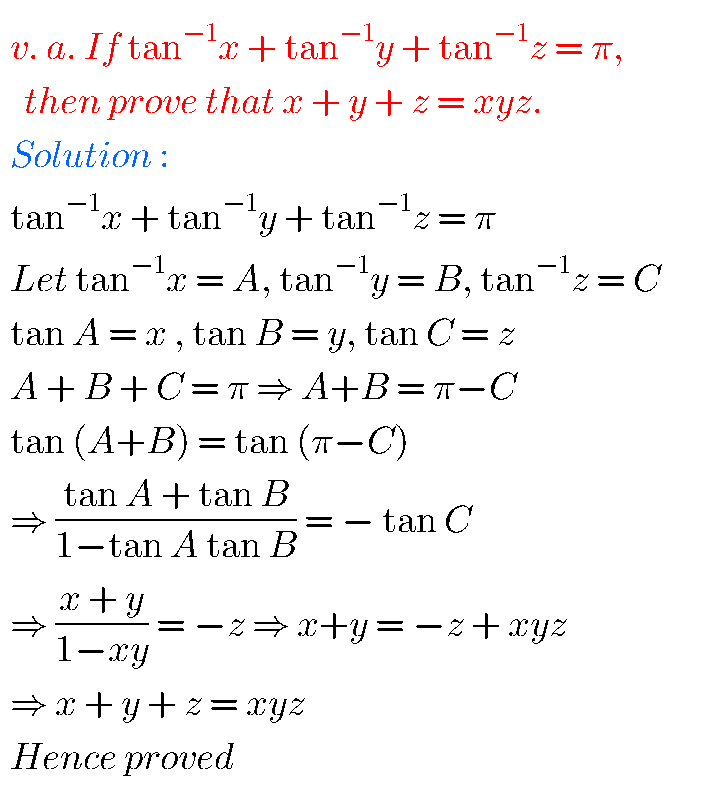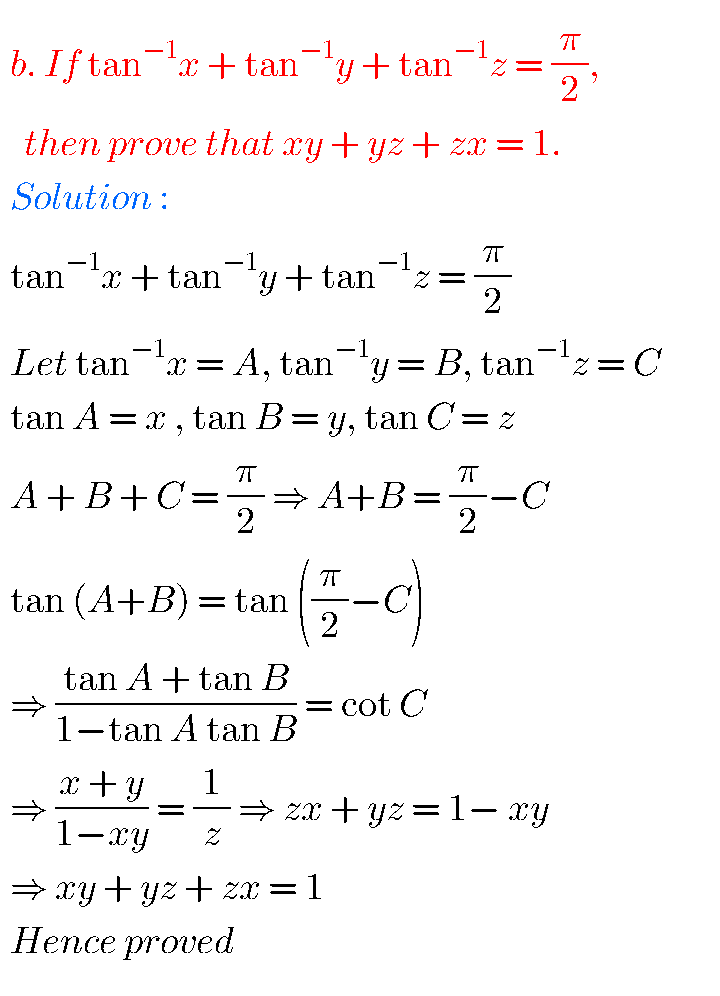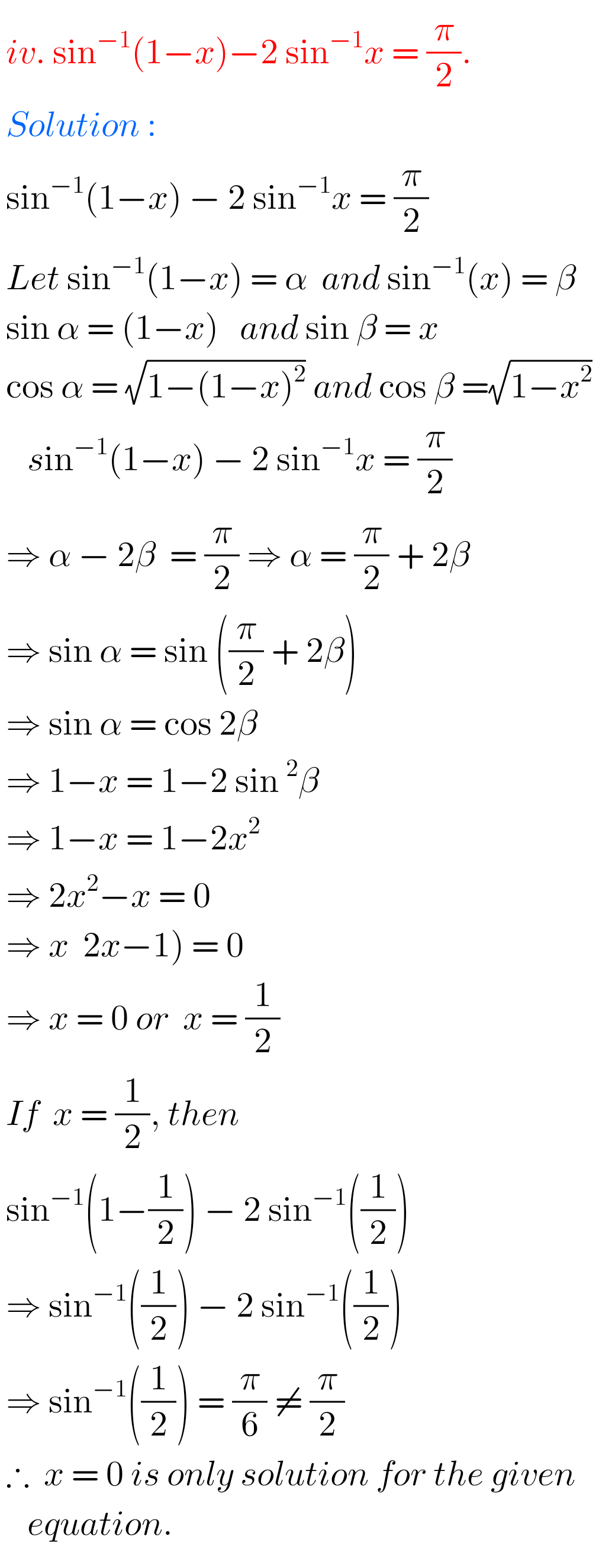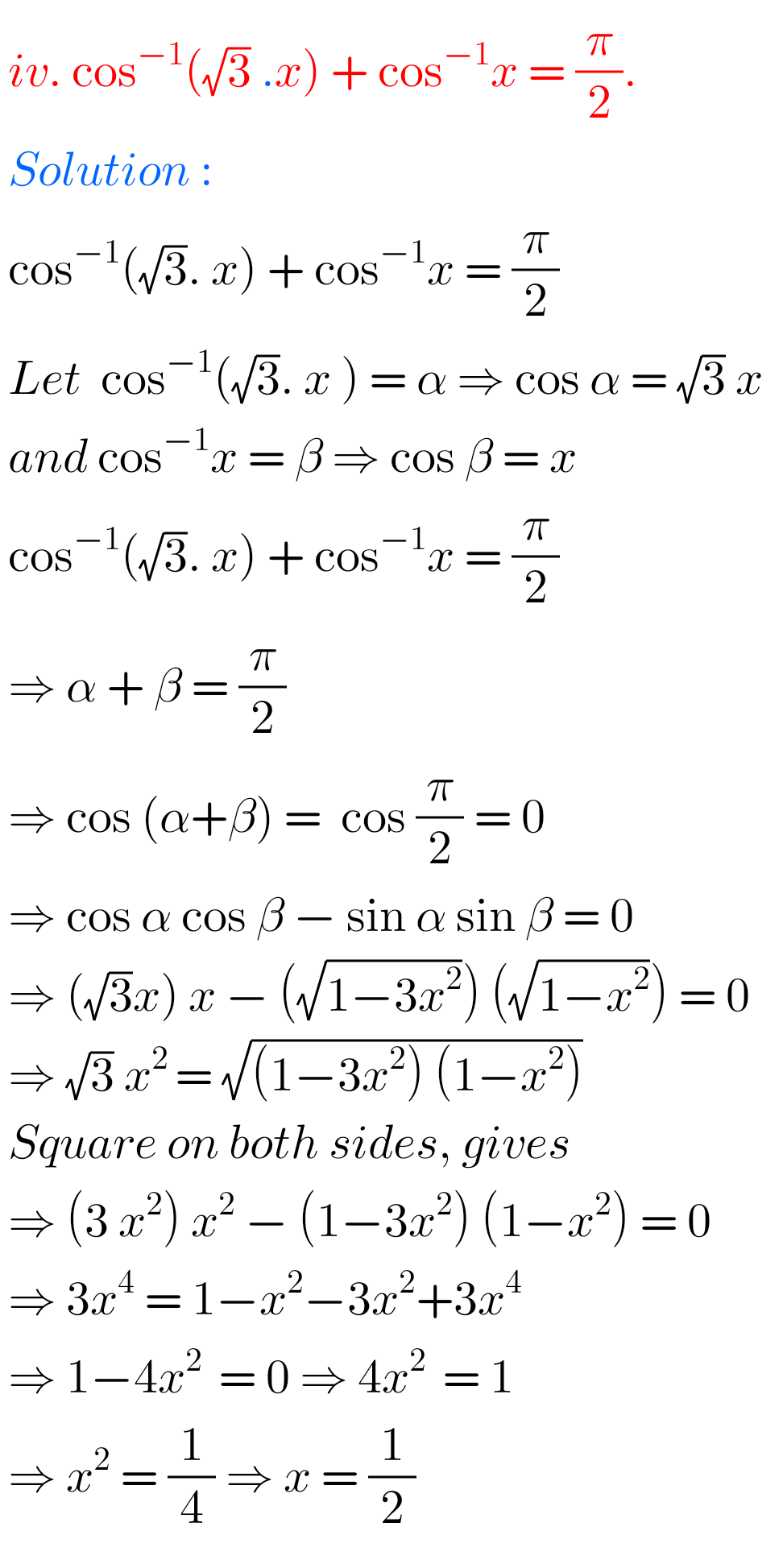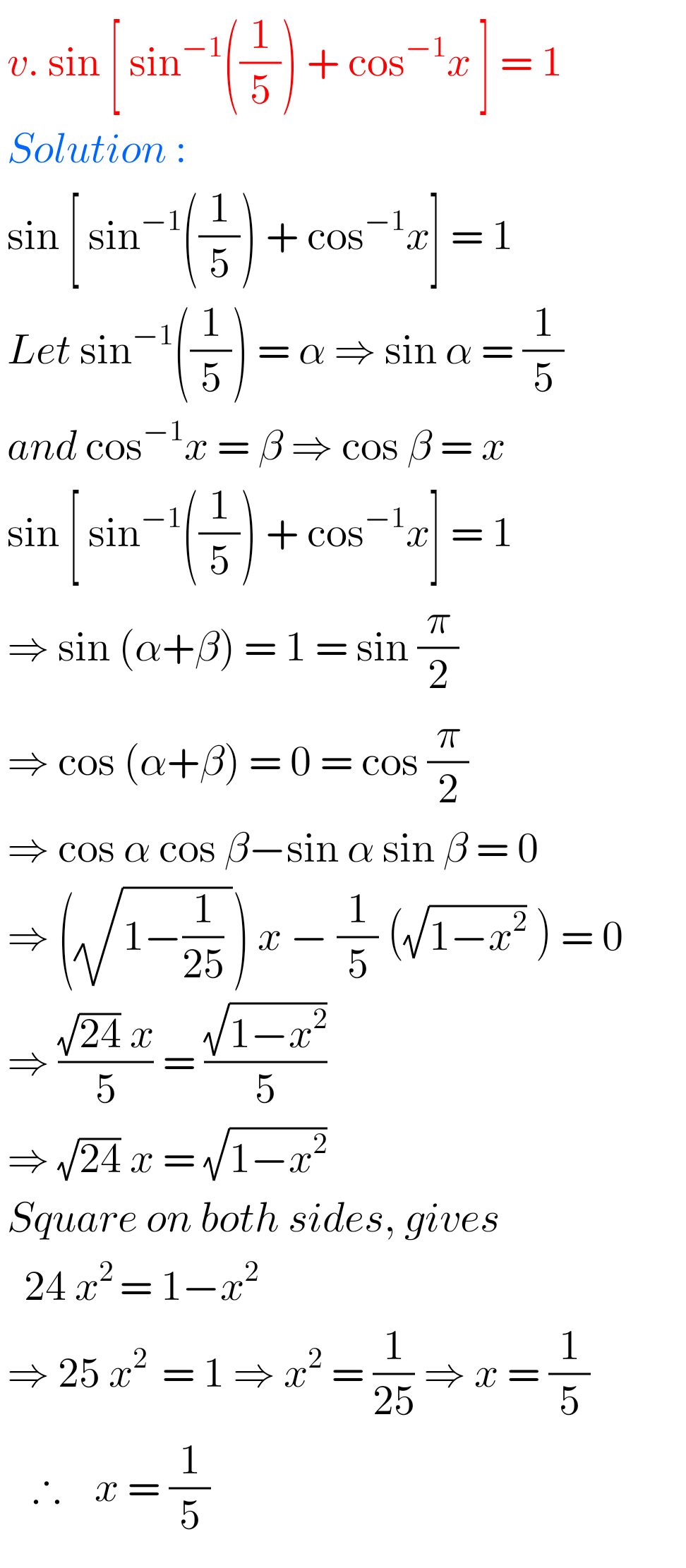Note : Observe the solutions and try them in your own method.

SSC Maths Solutions class 10

Inter Maths 1A solutions

Inter Maths 1B solutions

Inter Maths IIA text book solutions

Inter Maths II B text book solutions

You can see the solutions for text book Maths 1A

Functions

Exercise 1(a)

Exercise 1(b)

Exercise 1(c)

Mathematical Induction

Exercise 2(a)

Trigonometric Ratios up to Transformations

Exercise 6(a)

Exercise 6(b)

Exercise 6(c)

Exercise 6(d)

Exercise 6(e)

Exercise 6(f)

Trigonometric Equations

Exercise 7(a)

Inverse Trigonometric Equations

Exercise 8(a)

Hyperbolic Functions

Exercise 9(a)

Properties of Triangles

Exercise 10(a)

Exercise 10(b)

Maths 1B solutions for

Transformation of Axes

Exercise 2(a)

Limits and Continuity

Exercise 8(a)

Exercise 8(b)

M